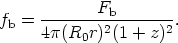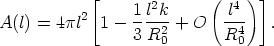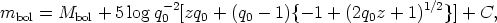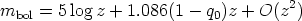Annu. Rev. Astron. Astrophys. 1988. 26: 561-630 Copyright © 1988 by Annual Reviews. All rights reserved

### 4. THE REDSHIFT-MAGNITUDE EQUATION

The N(z, q0) volume-redshift relation of the last section is very difficult to apply in practice because complete galaxy counts in redshift space require redshift measurements of every galaxy (of all types and surface brightness) in the volume, or else a correction for sample incompleteness in a redshift survey that is complete to a given magnitude limit (Loh & Spillar 1986, Loh 1986). These corrections must be highly precise if the small differences in the N(m, q0) curves in Figure 1 are to be measured. For this reason, the value of q0 via this route is quite uncertain at the moment.

The easier test observationally is the count-magnitude relation, N(m, q0), used in its most elementary form by Hubble (1936b), following the theory set out by Hubble & Tolman (1935) for N(r). We now cast their discussion into modern form by using the closed equation for the apparent magnitude-redshift relation via the Mattig equations and thereby changing N(z, q0) into the N(m, q0) count-magnitude prediction.

The apparent bolometric flux fb received at Earth from a galaxy receding with redshift z whose absolute flux (at the source) is Fb was shown by Robertson (1938) (after some debate) to be(32)

For an appreciation of this equation, consider a sphere of interval radius l (Equations 12, 13) centered on the source, over which the flux of a light pulse is spread at the time of light reception, t0, at the Earth. The area of this sphere is not 4l2 if the geometry is non-Euclidean but is, rather, 4(R0r)2, where R0r = R0sin l /R using Equation 12 for k = + 1. As in the case of the spherical cap of Equation 5, this area is smaller than 4l2 owing to the spatial curvature if k = + 1, or larger if k = - 1:The difference in the area compared with the Euclidean case is accounted for in Equation 32 by the (R0r)2 factor rather than by simply using the interval distance l, which would be incorrect. The (1 + z)2 term accounts for the energy depletion and dilution factors of the radiation due to the redshift. One factor arises because each photon is decreased in energy by (1 + z), and hence the entire ensemble is depleted by the same factor. The second factor of (1 + z) is present if the redshift is due to true expansion. It is caused by the increased path length, with the consequent decrease in the energy density. If the Universe is not expanding, the second (1 + z) factor would not be present, a crucial point for the surface brightness test discussed in Section 8.

Converting Equation 32 into magnitudes and using Equation 30 for (R0r)2 gives the theoretical m(z, q0) equation for the Hubble diagram in terms of the bolometric magnitude:(33)

where the constant C is 2.5 log 4+ 5 log c/H0. Note that the factor (1 + z)2 of Equation 32 is incorporated in Equation 33 as part of the theory. Some earlier writers, following Hubble, included the -5 log(1 + z) factor as a correction term to the observed magnitudes (as part of a generalized K term). This is not the modem practice, however, which, as done here, carries this factor into Equation 33 via Equation 32. This point is very important if the reader is to understand Hubble's (1936b) method of correction, which differs fundamentally from the modem practice, based on the equations given here.

Series expansion of Equation 33 gives the well-known equation (e.g. Robertson 1955, McVittie 1956)(34)

used by Humason et al. (1956; hereinafter HMS) in their early analysis of cluster data. Although adequate to z ~ 0.3, the deviations of Equation 34 from Equation 33 for larger z become inadequately large (cf. Mattig 1958, his Figure 1).